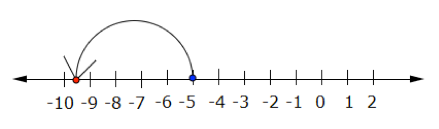### Home > CC1 > Chapter Ch4 > Lesson 4.1.2 > Problem4-20

4-20.

Each expression below begins with $-5$ and then adds something to it. As you look at each expression, state which direction you should move on a number line if you start at $-5$. Then simplify each expression.

For example, if the expression reads $-5+(-9)$, you would write “left, $-14$,” since from $-5$ you would move left on a number line $9$ units and would end up at $-14$.

1. $-5+(-4.5)$

See the diagram below.

1. $-5+(-8)$

See the hint for part (a).

Left, $-13$.

1. $-5+6\frac{3}{5}$

Which direction should you move to add